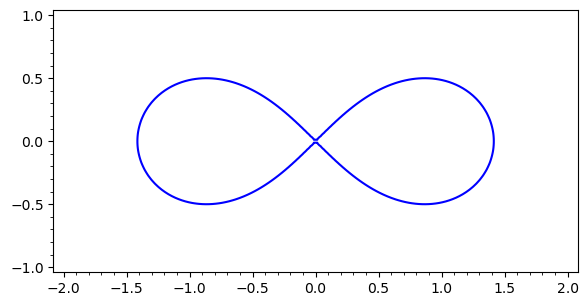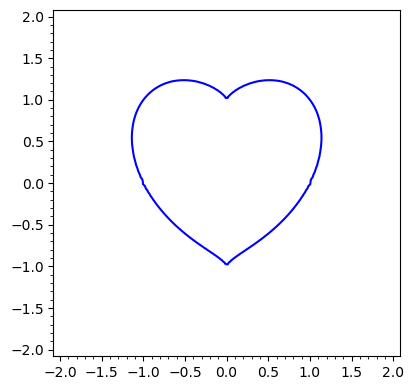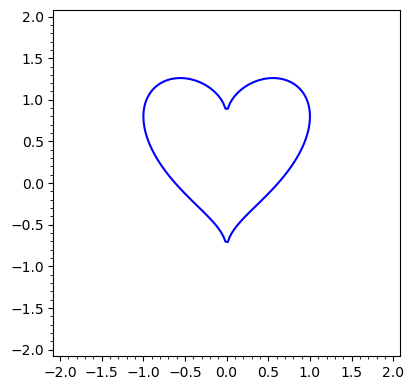# Lemniskate (Bernoulli)¶

In :
var("x y")
F(x,y)=(x^2+y^2)^2-2*(x^2-y^2)
implicit_plot(F(x,y), (x,-2,2), (y,-1,1))

Out:In :
show(F.gradient())


# Heart functions¶

In :
var("x y")
G(x,y)=(x^2+y^2-1)^3-x^2*y^3
implicit_plot(G(x,y), (x,-2,2), (y,-2,2))

Out:In :
var("x y")
H(x,y)=(5*y/4  -  sqrt(abs(x)))^2+x^2-1
implicit_plot(H(x,y), (x,-2,2), (y,-2,2))

Out:# Skew-symmetric linear differential equations¶

Careful here the computer makes mistakes when trying to solve the differential equations for long intervals!

In :
var('x,y,z,t')
ode_rhs = [-y-t*z, x,t*x]
p=desolve_system_rk4(ode_rhs,ics=[-10,0,1,1],vars=[x,y,z], ivar=t, end_points=10, step=0.05)
q=[[x,y,z] for t,x,y,z in p]
line3d(q, color="blue")+ implicit_plot3d(x^2+y^2+z^2==1.9, (x,-2,2), (y,-2,2), (z,-2,2), color='plum')

Out: